﻿ 直升机后缘襟翼驱动器迟滞现象仿真与抑制Download PDF文章快速检索 高级检索

Hysteresis modeling and suppression of piezoelectric actuator for helicopter trailing-edge flaps
ZHOU Jinlong, DONG Linghua, YANG Weidong
National Key Laboratory of Science and Technology on Rotorcraft Aeromechanics, College of Aerospace Engineering, Nanjing University of Aeronautics and Astronautics, Nanjing 210016, China
Abstract: Piezoelectric actuators are used to drive trailing-edge flaps of helicopters, but hysteresis of these actuators can degrade the vibration control performance of the active rotor. To solve this problem, hysteresis modeling and suppression are studied for a piezoelectric actuator. The hysteresis curves of this actuator at different frequencies are measured, and the Bouc-Wen model is utilized to model its rate-dependent hysteresis. Particle Swarm Optimization (PSO) algorithm is selected to identify the unknown parameters of the Bouc-Wen model, and a good agreement is shown between experimental results and model outputs for a range of frequencies from 10 Hz to 60 Hz, demonstrating that the established model is capable of simulating the actuator's dynamic hysteresis. A compound control scheme combining feedforward control based on inverse Bouc-Wen model and PID feedback control is established, and experimental results show that hysteresis of the piezoelectric actuator is suppressed remarkably at frequency range of 10 Hz to 60 Hz. Hysteresis model of the piezoelectric actuator is incorporated into helicopter rotor dynamic model to study the effect of actuator hysteresis on vibration control performance. Simulations of a model rotor with trailing-edge flaps are conducted in moderate speed forward flight condition. The results show that hysteresis can result in performance degradation of the trailing-edge flaps, while the compound control algorithm has the potential to improve the control authority of active rotor.
Keywords: helicopter    rotor    trailing-edge flap    piezoelectric actuator    hysteresis

1 驱动器迟滞建模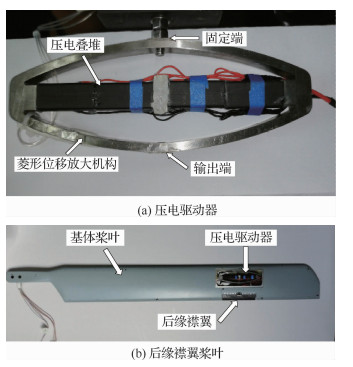图 1 压电驱动器与后缘襟翼桨叶 Fig. 1 Piezoelectric actuator and rotor blade with trailing-edge flap

 参数 数值 空载最大输出位移/mm 0.51 最大输出力/N 243 刚度/(N·mm-1) 550.2 质量/g 202 一阶共振频率/Hz 543.4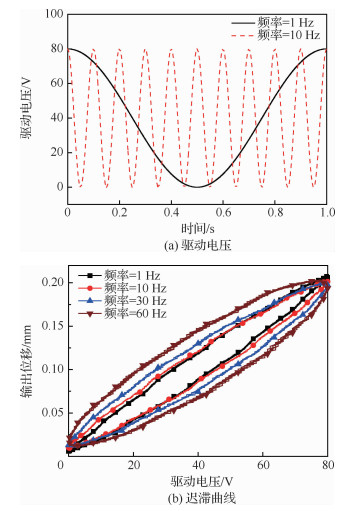图 2 不同频率下迟滞现象 Fig. 2 Hysteresis at different frequencies

 $\dot{h}=\alpha d \dot{u}-\beta|\dot{u}| h|h|^{n-1}-\gamma \dot{u}|h|^{n}$ （1）

 $\dot{h}=\alpha d \dot{u}-\beta|\dot{u}| h-\gamma\dot{u}|h|$ （2）

 $m \ddot{x}+b \dot{x}+k x=k(d u-h)$ （3）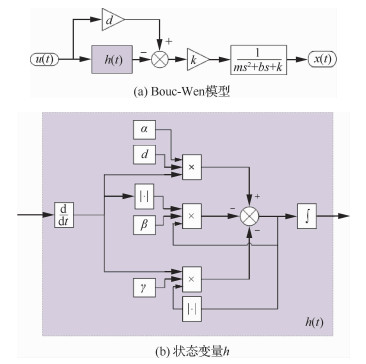图 3 Bouc-Wen模型框图 Fig. 3 Block diagram of Bouc-Wen model

 $f_{{\rm r}}=\frac{1}{2 \pi} \sqrt{\frac{k}{m}}$ （4）

 $m=\frac{k}{\left(2 \pi f_{\mathrm{r}}\right)^{2}}$ （5）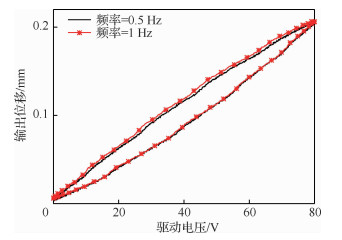图 4 低频迟滞 Fig. 4 Hysteresis at low frequencies

 $x=d u-h$ （6）

 $F(d, \alpha, \beta, \gamma)=\frac{1}{N} \sum\limits_{i=1}^{N}\left(x_{i}-\hat{x}_{i}\right)^{2}$ （7）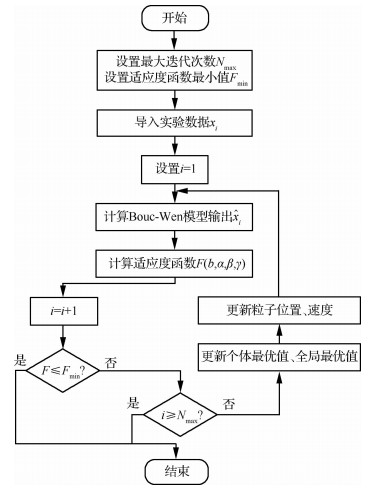图 5 粒子群算法辨识流程 Fig. 5 Flow chart of PSO identification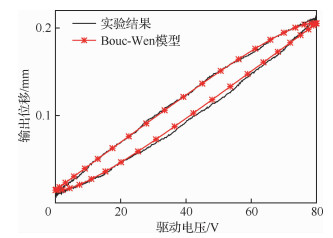图 6 实验结果与模型输出比较(1 Hz) Fig. 6 Comparison of experimental result and model output(1 Hz)

 参数 数值 m/kg 4.720×10-3 b/(N·s·m-1) 475.3 k/(N·m-1) 5.502×105 d/(mm·V-1) 2.7×10-3 α 3.893×10-1 β 1.0×10-1 γ -2.766×10-2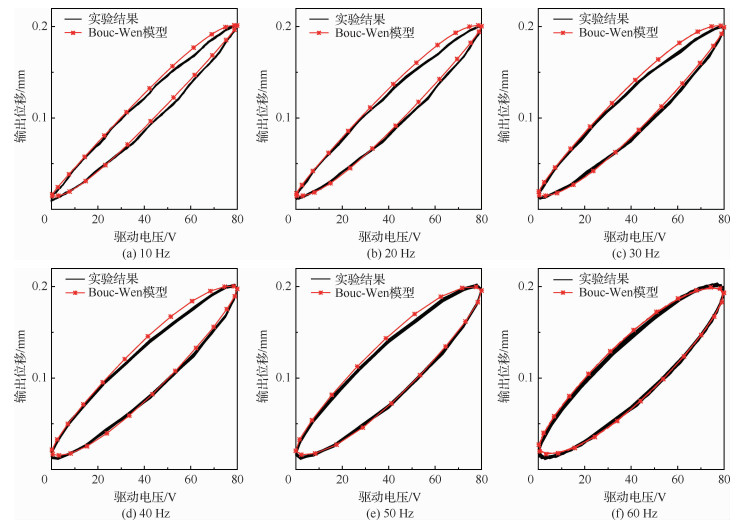图 7 不同频率下模型输出与实验结果比较 Fig. 7 Comparison of experimental results and model outputs at different frequencies
2 迟滞抑制

 $u=\frac{m}{k d} \ddot{x}+\frac{b}{k d} \dot{x}+\frac{1}{d} x+\frac{h}{d}$ （8）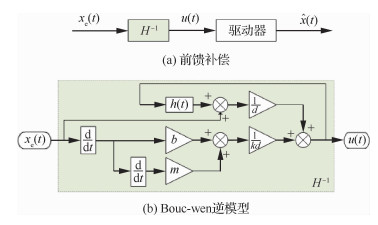图 8 前馈补偿 Fig. 8 Feedforward compensation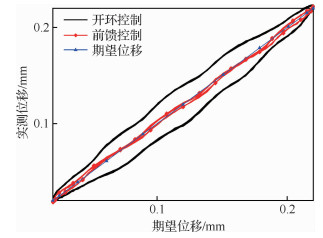图 9 前馈控制结果(10 Hz) Fig. 9 Results of feedforward control (10 Hz)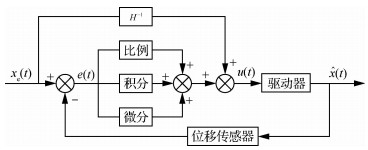图 10 复合控制结构 Fig. 10 Compound control scheme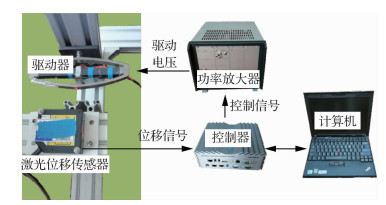图 11 实验装置 Fig. 11 Experiment setup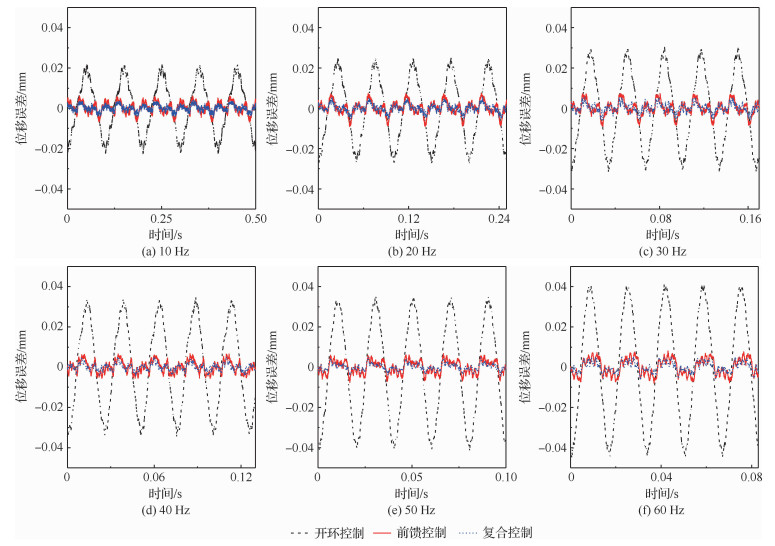图 12 位移误差 Fig. 12 Displacement error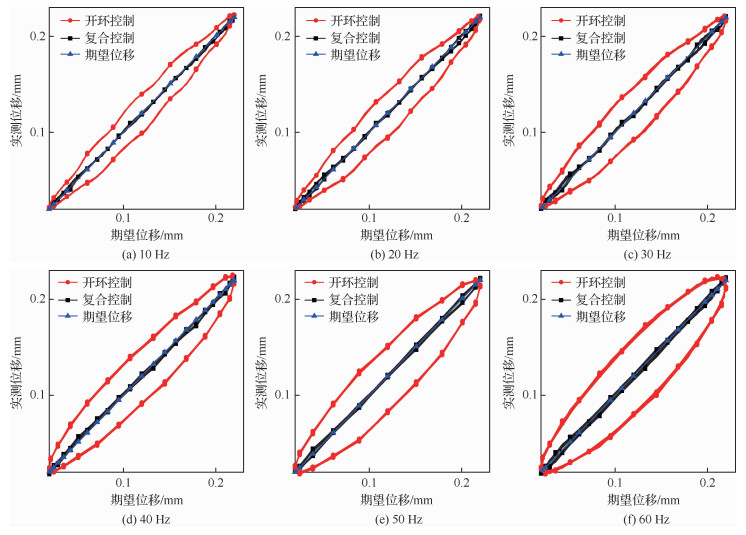图 13 复合控制效果 Fig. 13 Results of compound control
3 驱动器迟滞对振动控制性能影响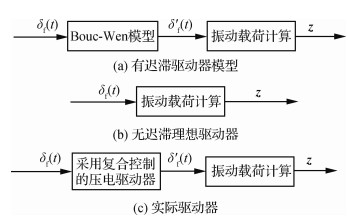图 14 驱动器有/无迟滞状态下振动载荷计算 Fig. 14 Vibration load calculations of actuator with and without hysteresis

 $\int_{t_{1}}^{t_{2}}(\delta U-\delta T-\delta W) \mathrm{d} t=0$ （9）

 $\delta U=\sum\limits_{i=1}^{i=N_{\mathrm{b}}}\left(\delta U_{\mathrm{b} i}+\delta U_{\mathrm{f} i}\right)$ （10）
 $\delta T=\sum\limits_{i=1}^{i=N_{\mathrm{b}}}\left(\delta T_{\mathrm{b} i}+\delta T_{\mathrm{f} i}\right)$ （11）
 $\delta W=\sum\limits_{i=1}^{i=N_{\mathrm{b}}}\left(\delta W_{\mathrm{b} i}+\delta W_{\mathrm{f} i}\right)$ （12）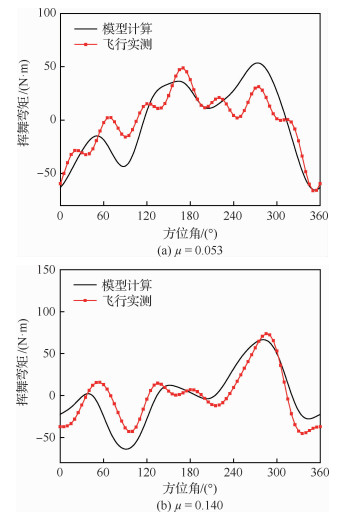图 15 模型计算结果与飞行实测数据 Fig. 15 Model calculation results and flight measurement datas

 $\boldsymbol{z}=\boldsymbol{T\theta}+\boldsymbol{z}_{0}$ （13）

 $J=\boldsymbol{z}^{\mathrm{T}} \boldsymbol{Qz} +\boldsymbol{\theta}^{\mathrm{T}} \boldsymbol{R} \boldsymbol{\theta}$ （14）

 $\frac{\partial J}{\partial \boldsymbol{\theta}}=0$ （15）

 $\boldsymbol{\theta}_{\mathrm{opt}}=\left(\boldsymbol{T}^{\mathrm{T}} \boldsymbol{Q} \boldsymbol{T}+\boldsymbol{R}\right)^{-1} \boldsymbol{T}^{\mathrm{T}} \boldsymbol{Q}\left(\boldsymbol{T} \boldsymbol{\theta}_{0}-\boldsymbol{z}_{0}\right)$ （16）

 参数 数值 桨叶片数Nb 3 旋翼半径R/m 5.25 桨叶弦长c/m 0.35 工作转速Ω/(r·min-1) 387 负扭转θ1/(°) -4.9 襟翼展向长度Lf/m 0.525 襟翼弦向长度cf/m 0.07 襟翼安装位置 0.70R-0.80R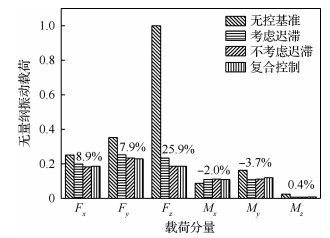图 16 考虑与不考虑迟滞情况下的振动控制效果 Fig. 16 Vibration control performances with and without hysteresis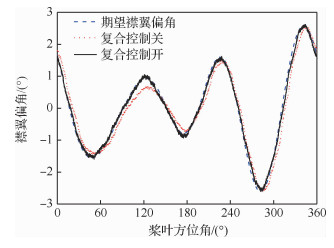图 17 襟翼偏角 Fig. 17 Trailing-edge flap deflection

4 结论

1) 压电驱动器在动态驱动电压作用下表现出的迟滞现象具有明显的率相关特性，随着驱动信号频率的升高，迟滞现象更加显著，仿真研究表明在中等速度稳态前飞状态下，驱动器迟滞会影响后缘襟翼振动控制效果。

2) 粒子群算法能够有效辨识Bouc-Wen模型参数，建立的Bouc-Wen模型能够在较宽的频率范围内较为精确地描述该压电驱动器的迟滞现象。

3) 采用基于Bouc-Wen逆模型的前馈控制与PID反馈控制的复合控制策略能够显著地抑制该压电驱动器的迟滞现象，驱动器位移输出误差控制在5 μm内，并且该控制策略在10~60 Hz频率范围内具有良好的适应性。

  STRAUB F K, ANAND V R, BIRCHETTE T S, et al. SMART rotor development and wind tunnel test: ARC-E-DAA-TN453[R]. Washington, D.C.: NASA, 2009.  RABOURDIN A, MAURICE J B, DIETERICH O, et al. Blue pulse active rotor control at airbus helicopters-new EC145 demonstrator and flight test results[C]//AHS 70th Annual Forum, 2014: 1-24.  DIETERICH O, RABOURDIN A, MAURICE J B, et al. Blue Pulse active rotor control by trailing edge flaps at airbus helicopters[C]//41st European Rotorcraft Forum, 2015: 1-13.  STRAUB F K, BYRNS J EV. Application of higher harmonic blade feathering on the oh-6a helicopter for vibration reduction: NASA Contractor Report 4031[R]. Washington, D.C.: NASA, 1986.  HABER A, JACKLIN S A, SIMONE G. Development manufacturing and component testing of an individual blade control system for a uh-60 helicopter rotor[C]//American Helicopter Society Aerodynamics, Acoustics, and Evaluation Technical Specialists Meeting, 2002: 1-10.  ARNOLD U T P. Recent flight test results from the ch-53g helicopter[C]//29th European Rotorcraft Forum, 2003: 1-15.  ARNOLD U T P, FURST D. Closed loop IBC results from ch-53g flight tests[J]. Aerospace Science and Technology, 2005, 9(5): 421-435. Click to display the text  HALL S R, PRECHTL E F. Preliminary testing of a Mach-scaled active rotor blade with a trailing-edge servo-flap[C]//Smart Structures and Materials 1999: Smart Structures and Integrated Systems, 1999: 14-21.  PRECHTL E F, HALL S R. Closed-loop vibration control experiments on a rotor with blade mounted actuation[C]//41st Structures, Structural Dynamics, and Materials Conference and Exhibit. Reston, VA: AIAA, 2000: 1714-1728.  ELIF A E, PFALLER R, DENECKE U, et al. Piezo active rotor blade-challenges and solutions[C]//American Helicopter Society 69th Annual Forum. Reston, VA: AIAA, 2013: 1-14.  LEE T, CHOPRA I. Design of piezostack-driven trailing-edge flap actuator for helicopter rotors[J]. Smart Materials and Structures, 2001, 10(1): 15-24. Click to display the text  LEE T, CHOPRA I. Design issues of a high-stroke, on-blade piezostack actuator for a helicopter rotor with trailing-edge flaps[J]. Journal of Intelligent Material Systems and Structures, 2000, 11(5): 328-342. Click to display the text  CROZIER P, LECONTE P, DELRIEUX Y, et al. Wind-tunnel tests of a helicopter rotor with active flaps[C]//32nd European Rotorcraft Forum, 2006: 1-16.  VISWAMURTHY S R, RAO A K, GANGULI R. Dynamic hysteresis of piezoelectric stack actuators used in helicopter vibration control:Experiments and simulations[J]. Smart Materials and Structures, 2007, 16(4): 1109-1119. Click to display the text  VISWAMURTHY S R, GANGULI R. Effect of piezoelectric hysteresis on helicopter vibration control using trailing-edge flaps[J]. Journal of Guidance, Control, and Dynamics, 2006, 29(5): 1201-1209. Click to display the text  VISWAMURTHY S R, GANGULI R. Modeling and compensation of piezoelectric actuator hysteresis for helicopter vibration control[J]. Sensors and Actuators A:Physical, 2007, 135(2): 801-810. Click to display the text  MALLICK R, GANGULI R, BHAT M S. An experimental and numerical study of piezoceramic actuator hysteresis in in helicopter active vibration control[J]. Journal of Aerospace Engineering, 2014, 228(5): 690-705. Click to display the text  MUIR E R, LIU L, FRIEDMANN P P, et al. Hysteresis characterization in piezoceramic stack actuator and its influence on vibration and noise reduction in helicopters using actively controlled flaps[C]//51st AIAA/ASME/ASCE/AHS/ASC Structures, Structural Dynamics, and Materials Conference. Reston, VA: AIAA, 2010: 1-20.  MUIR E R, LIU L, FRIEDMANN P P, et al. Effect of piezoelectric actuator hysteresis on helicopter vibration and noise reduction[J]. Journal of Guidance, Control, and Dynamics, 2012, 35(4): 1299-1311. Click to display the text  HASSANI V, TJAHJOWIDODO T, THANH N D. A survey on hysteresis modeling identification and control[J]. Mechanical Systems and Signal Processing, 2014, 49(1-2): 209-233. Click to display the text  CHANG C M, STRANO S, TERZO M. Modeling of hysteresis in vibration control systems by means of Bouc-Wen model[J]. Shock and Vibration, 2016, 2016: 1-14. Click to display the text  FUJII F, TATEBATAKE K I, MORITA K, et al. A Bouc-Wen model-based compensation of the frequency-dependent hysteresis of a piezoelectric actuator exhibiting odd harmonic oscillation[J]. Actuators, 2018, 7(3): 1-16. Click to display the text  杨晓京, 彭芸浩, 李尧. 压电微位移台的动态迟滞建模及实验验证[J]. 光学精密工程, 2016, 24(9): 2255-2261. YANG X J, PENG Y H, LI Y. Dynamic hysteresis modeling and experimental verification of piezoelectric positioning stage[J]. Optics and Precision Engineering, 2016, 24(9): 2255-2261. (in Chinese) Cited By in Cnki | Click to display the text  刘士明, 杨卫东, 虞志浩, 等. 带后缘小翼的旋翼振动载荷计算[J]. 航空动力学报, 2016, 31(6): 1496-1503. LIU S M, YANG W D, YU Z H, et al. Vibratory loads prediction of rotor with trailing edge flaps[J]. Journal of Aerospace Power, 2016, 31(6): 1496-1503. (in Chinese) Cited By in Cnki | Click to display the text  周金龙, 董凌华, 杨卫东, 等. 基于加权最小二乘法辨识的后缘襟翼智能旋翼振动载荷闭环控制仿真研究[J]. 振动与冲击, 2019, 38(4): 237-244. ZHOU J L, DONG L H, YANG W D, et al. Closed-loop vibration control simulation of helicopter active rotor with trailing-edge flaps based on weighted-least-squares-error identification method[J]. Journal of Vibration and Shock, 2019, 38(4): 237-244. (in Chinese) Cited By in Cnki | Click to display the text  HEFFERNAN P M, GAUBERT M. Structural and aerodynamic loads and performance measurements of an SA349/2 helicopter with an advanced geometry rotor: NASA-TM-88370[R].Washington, D.C.: NASA, 1986.
http://dx.doi.org/10.7527/S1000-6893.2019.23384

0

#### 文章信息

ZHOU Jinlong, DONG Linghua, YANG Weidong

Hysteresis modeling and suppression of piezoelectric actuator for helicopter trailing-edge flaps

Acta Aeronautica et Astronautica Sinica, 2020, 41(4): 223384.
http://dx.doi.org/10.7527/S1000-6893.2019.23384